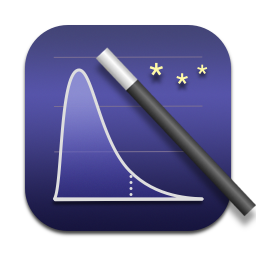# In Praise of Small Data

October 20, 2012

Lately there has been a lot of talk about the “Big Data” (petabytes and so forth) and how much valuable information is just waiting to be extracted from it.

One might think that analyzing more data is better than analyzing less data. Often, this is true. More often, it is not.

There are diminishing returns to the amount of information you can extract from data. The tenth gigabyte is worth much less than the second gigabyte. The hundredth gigabyte is worth less than than the tenth.

How much less? In regression analysis, the information you can derive from a data set is related to the square root of the size of the data set. That is, if you double the size of your data set, you only glean about 40% more information. On its face, a hundred gigabytes seems like it should have a hundred times more information than a single gigabyte, but from the standpoint of statistics, it only has ten times as much. So the hundredth gigabyte has about a tenth of the informational value as the second gigabyte.

And then there are diminishing returns to the value of information itself. Suppose you wish to estimate the effectiveness of a new drug (or something). Suppose the actual effect is 1.53824, pick whatever units you like. You might be able to make a decision just knowing the first decimal place (that is, you estimate the effect around 1.5). The second decimal place might be nice to have, but unless you are working in the physical sciences, it is unlikely that knowing the fifth decimal place to be a 4 instead of an 8 will have any effect on anyone’s perception of reality.

Taken together (diminishing information from data, diminishing decision-making value from information), the marginal decision-making value of data drops rapidly. Which is to say, a majority of the decision-making value from “Big Data” can be gleaned from just a small subset.

In fact, that is the entire point of statistics — figuring out how much information can be derived from a sample of data randomly chosen from a larger population. If your goal is to answer questions such as “How does this number vary across these groups?”, “Are these two variables correlated?”, or “What is the effect of X on Y after controlling for A, B, C, D, and E?”, you can get precise answers just by looking at small samples of data, for the same reason that marine scientists study the ocean by putting a few droplets of water under a microscope.

And there is a cost to analyzing Big Data. The amount of time it takes to run a regression analysis on a data set is proportional to the number of rows in the data set. Time has a cost; you must pay for computational resources, and you must pay a person to sit and wait for an answer. So while the value of data grows sub-linearly (in the best case, in proportion to the square root), the cost grows linearly. Worse, you may lose a strategic advantage while you wait for analyses to be completed. That cost is difficult to quantify or predict.

You might think you need to analyze Big Data if you are detecting outliers or searching for a needle in a haystack (fighting terror, catching fraud, discovering the next Jeremy Lin). Actually, you can usually use a small sample while you build and test your predictive models, then you can run the best model against Big Data and see which rows appear to be out-of-the-ordinary. It’s often a good idea to use this approach because the time required to estimate a model is proportional to the square of the number of parameters, but the time required to apply a model is only linear in the number of parameters. That is, if your model has 10 parameters, you pay for each row 100 times while you are estimating the model, but you only pay for each row 10 times while you are applying the model. So where computation time is a concern, it often makes sense to build models against small data, and run them on Big Data later.

Analyzing Big Data is usually only necessary if you have a model with a large number of parameters to be estimated; predicting preferences and analyzing language come to mind (and for the reasons mentioned above, estimating a large number of parameters on Big Data gets quite expensive). These models are usually associated with data-driven applications rather than analytic insight. If you have a small number of parameters in your model — that is, if you are only interested in discovering a few key numbers, which is almost always the case in business and the social sciences — small data will do just fine.

In fact, if you are running a randomized experiment, you will usually have just one parameter in your model (control versus treatment). In that case a few hundred observations is usually more than necessary. A thousand observations is overkill. You don’t need Big Data; you need a spreadsheet.

So unless you are building an application with thousands or millions of parameters in the model — which is to say, unless you really know what you’re doing — Big Data should not be seen as a source of value. Instead, it should be seen as a source of costs. Most of these costs can be eliminated, and most of the value preserved, by understanding statistics.

You’re reading evanmiller.org, a random collection of math, tech, and musings. If you liked this you might also enjoy: